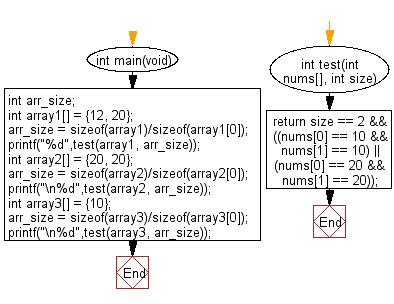﻿ C exercises: Check a given array of integers and return true if the array contains 10 or 20 twice - w3resource# C Exercises: Check a given array of integers and return true if the array contains 10 or 20 twice

## C-programming basic algorithm: Exercise-43 with Solution

Write a C program to check a given array of integers and return true if the array contains 10 or 20 twice. The length of the array will be 0, 1, or 2.

C Code:

``````#include <stdio.h>
#include <stdlib.h>
int main(void){
int arr_size;
int array1[] = {12, 20};
arr_size = sizeof(array1)/sizeof(array1);
printf("%d",test(array1, arr_size));
int array2[] = {20, 20};
arr_size = sizeof(array2)/sizeof(array2);
printf("\n%d",test(array2, arr_size));
int array3[] =  {10};
arr_size = sizeof(array3)/sizeof(array3);
printf("\n%d",test(array3, arr_size));
}
int test(int nums[], int size)
{
return size == 2
&& ((nums == 10 && nums == 10)
|| (nums == 20 && nums == 20));
}
``````

Sample Output:

```0
1
0
```

Pictorial Presentation:Flowchart:C Programming Code Editor: